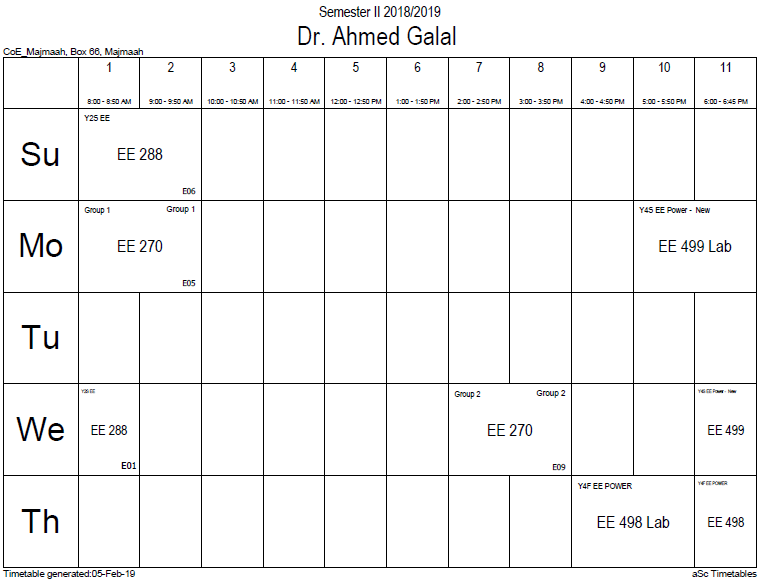## Uncertainty princi

In quantum mechanics, the uncertainty principle is any of a variety of mathematical inequalities asserting a fundamental lower bound on the precision with which certain pairs of physical properties of a particle, such as position x and momentum p, can be simultaneously known. The more precisely the position of some particle is determined, the less precisely its momentum can be known, and vice versa. The original heuristic argument that such a limit should exist was given by Werner Heisenberg in 1927. A more formal inequality relating the standard deviation of position σx and the standard deviation of momentum σp was derived by Kennard later that year (and independently by Weyl in 1928),$sigma_{x}sigma_{p} geq frac{hbar}{2},$

where ħ is the reduced Planck constant.

Historically, the uncertainty principle has been confused with a somewhat similar effect in physics, called the observer effect, which notes that measurements of certain systems cannot be made without affecting the systems. Heisenberg himself offered such an observer effect at the quantum level (see below) as a physical "explanation" of quantum uncertainty. However, it has since become clear that quantum uncertainty is inherent in the properties of all wave-like systems, and that it arises in quantum mechanics simply due to the matter wave nature of all quantum objects. Thus, the uncertainty principle actually states a fundamental property of quantum systems, and is not a statement about the observational success of current technology.

Mathematically, the uncertainty relation between position and momentum arises because the expressions of the wavefunction in the two corresponding bases are Fourier transforms of one another (i.e., position and momentum are conjugate variables). A similar tradeoff between the variances of Fourier conjugates arises wherever Fourier analysis is needed, for example in sound waves. A pure tone is a sharp spike at a single frequency. Its Fourier transform gives the shape of the sound wave in the time domain, which is a completely delocalized sine wave. In quantum mechanics, the two key points are that the position of the particle takes the form of a matter wave, and momentum is its Fourier conjugate, assured by the de Broglie relation$p=hbar k$, where$k$ is the wavenumber.

In the mathematical formulation of quantum mechanics, any pair of non-commuting self-adjoint operators representing observables are subject to similar uncertainty limits. An eigenstate of an observable represents the state of the wavefunction for a certain measurement value (the eigenvalue). For example, if a measurement of an observable$A$ is taken then the system is in a particular eigenstate$Phi$ of that observable. The particular eigenstate of the observable$A$ may not be an eigenstate of another observable$B$. If this is so, then it does not have a single associated measurement as the system is not in an eigenstate of the observable.

### Office HoursNo office hours### My Timetable### Contactsemail: [email protected]

Phone: 2570

### Welcome

Welcome To Faculty of Engineering### IEEEhttp://www.ieee.org/

/

### Bookmarkshttp://www.utk.edu/research/

http://science.doe.gov/grants/index.asp

http://www1.eere.energy.gov/vehiclesandfuels/

http://www.eere.energy.gov/

### Upcoming Conferences### Engineering quotes### Travel Web Siteshttp://www.hotels.com/

http://www.orbitz.com/

http://www.hotwire.com/us/index.jsp

http://www.kayak.com/

### Blackboardستقام اختبارات الميدتيرم يوم الثلاثاء 26-6-1440

حسب الجدول المعلن بلوحات الاعلان

### Summer trainingThe registration for summer training will start from 5th week of second semesterClass registration week 1

### برنامج التجسير### إحصائية الموقع

عدد الصفحات: 2879

البحوث والمحاضرات: 1280

الزيارات: 99543